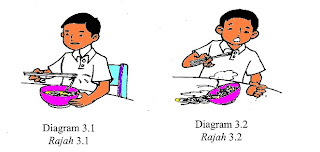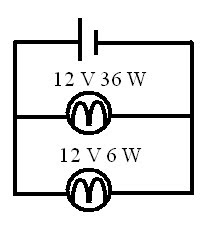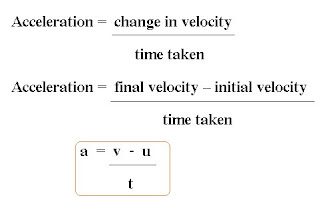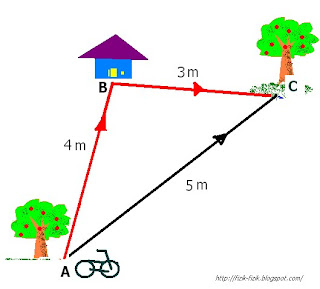## Friday, October 14, 2011

### Percubaan SPM Selangor fizik Kertas 1 2011

Selangor fiz K1 2011

## Saturday, September 24, 2011

### soalan percubaan SPM Selangor. Fizik kertas 3 berjawapan

sesiapa yang ada soalan negeri lain tolong kongsi bersama saya, saya akan upload kan soalan kertas 2 and 1 selepas 29 sep 2011.

Selangor Phy p3 Wit Ans

## Saturday, September 17, 2011

### Interference and refraction

Diagram below shows the interference pattern of water waves.
Rajah dibawah menunjukkan corak interferen gelombang air.

What happens to the separation between  two  consecutive nodal lines, x,  if the
depth  of  water in the ripple tank is increased?
Apakah yang akan berlaku kepada jarak pemisahan 2 garisan nodal berturutan, x,  jika
kedalaman air dalam  tangki riak meningkat?

A.  Unchanged / Tidak berubah
B.  Increase / Bertambah
C.  Decrease / Berkurang

*tips: apply refraction of water wave.

## Friday, September 16, 2011

### Archimedes' Principle (calculation)

Pengubahsuain Soalan ke-15 Peperiksaan Percubaan SPM SBP 2011

1. A metal block is immersed in unknown liquid as shown in diagram below. If the liquid pressure exerted on top, P1 and at the bottom, P 1 are 1.0 x 105 Pa and 1.2 x 105 Pa respectively.
Satu bongkah logam direndamkan ke dalam sesuatu cecair seperti ditunjukkan rajah dibawah. Jika tekanan cecair dikenakan pada permukaan atas, P1 dan permukaan bawah, P2 adalah masing-masing 1.0 x 105 Pa and 1.2 x 105 Pa.

Calculate the buoyant force acting on the metal block?
Hitung daya julangan yang dikenakan ke atas bongkah logam itu?

Ans: 400N

2. A metal block is immersed in water as shown in diagram above.
Satu bongkah logam direndamkan ke dalam air seperti ditunjukkan rajah dibawah.

Calculate the buoyant force acting on the metal block?
Hitung daya julangan yang dikenakan ke atas bongkah logam itu?

Ans: 80 N

## Wednesday, August 10, 2011

### Archimedes Principle

Archimedes’ Principle state that “ When an object is immersed in a fluid (a liquid or a gas) ,the buoyant force
( upthrust force) on the object  is equal  to the weight of fluid displaced by the object.

From  Archimedes’s Principle :

Buoyant Force, Fb  = Weight of fluid displace
=  mg                 (note : F = ma)
=  ρVg               (note : ρ = m/V  )

Thus     Fb     =   ρ V g

Where  Fb   =  Bouyant Force or Upthrust
ρ     =  Density of fluid
V     =  Volume of fluid displaced or the volume of the object that immersed in the fluid.

Equation for buoyant force

1. Buoyant force = Weight of fluid displaced
2. Buoyant force = ρVg
3. Buoyant force = Weight object in air – weight in water

## Sunday, August 7, 2011

### Actual weight and apparent weight

Buoyant force makes thing seem to be lighter.

The weight of an object in air is its actual weight
The weight measured when the object is immersed in a fluid is its apparent weight.
The buoyant force is equal to the apparent loss in weight.
The object displaces a volume of water.
The bigger the volume of the object immersed, the bigger the volume of the liquid displaced.
•  Volume of liquid displaced  = volume of the submerged part of the  object.

Work example :

An object weights 2.5 N.  When it is fully submerged in a liquid, its apparent weight is 2.2 N.

(a) What is the buoyant  force on the object in  water?
Tips : Fb= Wc- Wa

(b) What is the weight of water displaced by the object?
Tips : Weight , W = mass, m x gravitational force, g
In SPM take g = 10 Nkg-1

(c) Determine the volume  of water displaced by  the object.
[density of water = 1000 kgm-3]
Tips : Density = mass / volume

(d) What is the volume of the object?
Tips : Volume of liquid displaced  = volume of the submerged part of the  object.

## Friday, August 5, 2011

### Buoyant Force

An object immersed in water is subjected to two forces:
a. the weight of the object which acts downwards, (It pushes the object down)
b. an up-thrust or buoyant force which acts upwards.(it pushes the object up)

If the force from the weight of the object is greater than the force from buoyancy, the object sinks.
Weight , W > Buoyant force, Fb ; object sink

If the buoyant force is greater than the force from the weight of the object, the object floats.

Weight , W < Buoyant force , Fb ; boject float

## Tuesday, June 14, 2011

### Jawapan fizik kertas 3 pertengahan tahun 2011

Marking Schemes Mid Term 2011 P3

## Monday, June 13, 2011

### Jawapan fizik kertas 2 tingkatan 5 ( pertengahan tahun )

sila semak jawapan anda dan jumpa saya esok untuk sebarang pembetulan .

answer phyiscs paper 2 form 5 ( midterm 2011)

## Friday, June 10, 2011

### Free fall

Objects fall because they are pulled towards the Earth by the force of gravity.

This force is known as the pull of gravity or the earth’s gravitational force.

The earth’s gravitational force tends to pull everything towards its centre.

Free fall
An object is falling freely when it is falling under the  force of gravity  only.
Objects and people will experience weightlessness during free fall.

Examples of objects not in free fall:

1. A piece of paper does not fall freely because its fall is affected by air resistance.
2. Flying birds because there is also an additional force of lift.
Examples of objects in free  fall:

1. An object falls freely only in vacuum. The absence of air means there is no air resistance to oppose the motion of the object. In vacuum, both light and heavy objects fall freely. They fall with the same acceleration ie. The acceleration due to gravity, g.
2. In a falling elevator.
(gravitational acceleration,g  =  9.8 ms-2, in SPM often approximated g as 10 ms-2)

## Thursday, June 9, 2011

### s.wg of conductor and resistance

Diagram  6.1  and  Diagram  6.2  show  circuits  used  to  investigate  the  relationship between resistance and cross sectional area of a wire. Constantan wire of s.w.g. 36 and s.w.g 24 with same length are used.
Rajah 6.1 dan Rajah 6.2 menunjukkan  litar yang digunakan untuk menyiasat   hubungan antara rintangan dan luas keratan rentas dawai. Dawai konstantan s.w.g. 36 dan s.w.g 24 dengan panjang yang sama digunakan.

(a)  Using Diagram 6.1 and Diagram 6.2;
Dengan menggunakan  Rajah 6.1 dan Rajah 6.2;

(i)  Compare the cross sectional area of the constantan wires .
Bandingkan luas keratan rentas dawai konstantan.

(ii) Compare the potential difference across the constantan wires.
Bandingkan beza keupayaan melalui dawai konstantan.

(iii) Compare the magnitude of the current that flow  along the constantan wires.
Bandingkan magnitud  arus yang mengalir melalui dawai-dawai  konstantan tersebut.

Dengan menggunakan jawapan anda dalam 6(b);

(i)  Compare the resistance of the  constantan wires.
Bandingkan rintangan dawai konstantan.

(ii) Relate the cross sectional area of constantan wire to the resistance of the wire.
Hubungkait luas keratan rentas dawai konstantan dengan  rintangan dawai.

(c)   What will happen  to the  reading of voltmeter when  another constantan wire s.w.g  36 of the same length is connected parallel to the wire in circuit Diagram 6.1?  Explain your answer.
Apakah yang berlaku kepada bacaan voltmeter apabila dawai konstantan s.w.g 36 yang sama panjang dipasang selari dengan dawai dalam litar Rajah 6.1?  Jelaskan jawapan anda.

*sewaktu menjawab soalan sebegini, gunakan maklumat yang diberi dan memerhati gambarajah yang sediakan.
*Jawab dengan ayat ringkas .
* tambahan :
Apakah maksud s.w.g ?
http://en.wikipedia.org/wiki/Standard_wire_gauge

a.
i)   Cross sectional area in Diagram 6.2 is larger than in Diagram 6.1.
ii)  Potential difference 6.1 > 6.2
iii)  Current for both circuits are the same

b.
i)   Resistance 6.1 > 6.2
ii)  As the cross sectional area increases, resistance decreases.

c) Reading of voltmeter decreases because total/effective resistance decreases.

## Wednesday, June 8, 2011

### Light bulb and resistanceDiagram shows a bulb labelled 12 V, 24 W.
a. What is meant be 12 V, 24 W.
b. Why filament is made of high resistance conductor.
c. State 2 factors that affected the resistance of the conductor (filament).

a. 12 V, 24 W means that the bulb will consume 24 J of electrical energy every second if it is connected to 12 V mains supply.

b. To give out more heat energy and the bulb will become brighter.
(If you connect a bulb to a constant current source, then its brightness increases with increasing resistance, because the power I2 R is proportional to the resistance)
c.
i) Length of  conductor
The resistance of a conductor increases with length.

ii) Cross sectional area ( thickness) of  conductor
The thicker the conductor, the lower the resistance.

iii) Temperature of conductor
Filament light bulbs are non Ohmic conductors,it resistance increases with temperature.
It is a known fact that as an filament light bulb gets hotter, it gets brighter as well.

iv) Material
For fixed length and thickness of a conductor, its resistance is affected by the type of material.
Example resistivity of tungsten is higher than aluminium.

## Saturday, May 7, 2011

### Selangor trial Paper 3 q3 (2011)~~heat

Diagram 3.1 shows a boy accidentally spilt a few drops of hot noodle soup onto his hand and felt slight pain. Later on, he accidentally spilt the whole bowl of hot noodle soup onto himself and felt extreme pain as shown in Diagram 3.2.

Rajah 3.1 menunjukkan mee sup panas terpercik ke atas tangan seorang budak lelaki secara tidak sengaja dan dia merasai sedikit kesakitan. Tidak lama kemudian, sekali lagi secara tidak sengaja dia menumpahkan keseluruhan mee sup yang panas itu ke atas dirinya dan merasai kesakitan yang amat sangat seperti ditunjukkan dalam Rajah 3.2.Based on the information and observation:
Berdasarkan maklumat dan pemerhatian tersebut:

(a)
State one suitable inference.
Nyatakan satu inferens yang sesuai.

(b)
State one hypothesis that could be investigated.
Nyatakan satu hipotesis yang boleh disiasat.

(c)
With the use of apparatus such as power supply, beaker, immersion heater and other apparatus, describe an experiment to investigate the hypothesis stated in 3(b).
In your description, state clearly the following:

Dengan menggunakan radas seperti bekalan kuasa, bikar, pemanas rendam dan lain-lain
radas, terangkan satu eksperimen untuk menyiasat hipotesis yang dinyatakan di 3(b).
Dalam penerangan anda, jelaskan perkara berikut:

(i)
The aim of the experiment.
Tujuan eksperimen.

(ii)
The variables in the experiment.
Pembolehubah dalameksperimen.

(iii)
list of apparatus and materials.
Senarai radas dan bahan.

(iv)
The arrangement of the apparatus.

(v)
The procedure used in the experiment.
Describe how to control the manipulated variable and how to measure the responding variable.
Prosedur yang digunakan dalam eksperimen.
Terangkan bagaimana mengawal pembolehubah dimanipulasikan dan bagaimana mengukur pembolehubah bergerak balas.

(vi)
The way to tabulate the data.
Cara untuk menjadualkan data.

(vii)
The way to analyse the data.
Cara untuk menganalisis data.

a. The heat/ time taken of the water depends on its mass/volume
b. The greater the mass/volume, the time taken increase

c .
Aim of the experiment
To investigate the relationship between the time taken and mass/volume

Variables in the experiment
Manipulated variable : mass/volume
Responding variable : Time taken
Constant variable : Initial temperature/specific heat capacity/ changes in temperature

List of apparatus and material
Power supply, thermometer, beaker, immersion heater, stirrer

Arrangement of apparatus

Controlling the manipulated variables
The 20 g of water is filled in the beaker.
The initial temperature, Ө 0, of water is recorded.
The heater is switched on until the water boiled.

Controlling the responding variables
The time taken for the water is observed and recorded/ The heat is calculated.

Repeat the experiment with four different values of mass.
Repeat with masses of boiling water 40 g, 60 g, 80 g and 100 g.

How you tabulate the data.

How you analyse the data.

## Monday, April 25, 2011

### Exercise Electrical Energy and Power1. Two light bulbs labelled as 12 V 36 W and 12 V 6 W respectively are connected in parallel to a 12 V car battery. What is the total amount of heat energy dissipated from the two bulbs in 15 seconds?

Ans : 630J

## Saturday, April 2, 2011

### March test 2011 answer scheme

Skeme Mar2011 Test

## Friday, March 18, 2011

### Acceleration and Deceleration (Retardation)

In the context of linear motion, the direction of the object in motion does not change; but the magnitude of the velocity may change.
When the velocity of an object changes, the object is said to be accelerating.

Acceleration is defined as the rate of change in velocity.Acceleration is a vector quantity. SI unit for acceleration, a is ms-2, metre per second squared.Positive acceleration
If the velocity of an object increases from an initial velocity, u, to a higher final velocity, v.
The acceleration of an object is uniform if its velocity increases at a uniform rate.

Negative acceleration is called deceleration.
If the velocity of an object decreases from an initial velocity, u, to a lower final velocity, v, its acceleration is negative.
The deceleration of an object is uniform if its velocity decreases at a uniform rate.

Zero acceleration
An object moving at a constant velocity, that is, the magnitude and direction of its velocity remain unchanged - is not accelerating; its acceleration is zero.

Quiz :

An object accelerates at a constant rate from rest and reaches a velocity of 10 ms-1 after 5.0 s.
What is it acceleration?

Tips : rest mean stationary ( zero velocity )

## Wednesday, March 2, 2011

### Speed of Sound

The speed of sound, v, in a medium can be determined using the formula v = fλ , whereλ is the wavelength and f is the frequency of the sound wave.

The SI units of v, f and λ are ms-1 , Hz and metre (m) respectively.

The speed of sound in solids is greater than the speed of sound in liquids which is greater than the speed of sound in gases.

The speed of sound is unaffected by pressure. If the atmospheric pressure changes, the speed of sound in air remains constant.

The speed of sound increases with temperature. At the peak of high-altitude mountains, the speed of sound is less than that at sea level. This is not due to the lower pressures but instead it is because of lower temperatures at the peak of the mountain.

## Monday, February 28, 2011

### Distance and displacementFigure shows a boy cycles from A to B, and then from B to C.

i ) Calculate the distance travelled by the boy and the displacement of the boy.

ii ) Calculate the speed and the velocity of the boy if the boy took 10 s to cycle from A to B and then to C.

iii) then the boy travelled back to A from C, calculate the total distance and displacement travelled by the boy.

Tips to answer this question :

Distance is the actual distance that was covered
Distance depends on the path, from point A --> B --> C in the figure.
Distance (symbol d) does not have a direction, so it is a scalar . It
always positive

Displacement (symbol s) is the shortest distance from the starting point to the endpoint.
Distance independent of path taken , from point A to C in the ﬁgure.
Displacement has direction and is therefore a vector. It can be positive or negative

Speed is rate of change of distance
. Speed is scalar quantity

Velocity is rate of change of displacement. Velocity vector quantity.

Distance
from A to B and then to is
3 m + 4 m
= 7 m

Displacement from A to B and then to C is 5 m.

Speed from A to B and then to C
7 m / 10 s
= 0.7 m/s

Velocity
from A to B and then to C is
5 m /10 s
= 0.5 m/s.

Distance from A-> B-> C -> B -> C is
3 m + 4 m + 3 m + 4 m
= 14 m

Displacement
from A-> B-> C -> B -> C is
5 m - 5 m
= 0 m
( Because the boy's location is the same as his starting location, we use negative sign to indicate opposite direction )